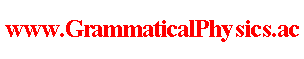since 2006 Help　Sitemap < Forum > < Definition > < Abstracts for the Meetings Held by the Physical Society > < JPS 2007 Spring Meeting > 28pSL-11New grammar version of Schrodinger equationUda's School / Yuuichi Uda---About a system with one degree of freedom, I want to find an equation obeyed by Φ in the new grammar, which represents a quantum history whose quantum state at time t is represented by wave function ψ(□,t) by the following functional Φ.Φ[χ]=exp[α∫dt φ(χ(t),t)]; ψ(x,t)= exp φ(x,t)・・・※As a clue, it seems plausible course to select the equation for Φ as it reduces to the ordinary schrodinger equation for ψ in the special case that ※ holds.Along this course, I tried making the following equation.(ih／2πα) lim (1／ε)(Φ[χ']－Φ[χ])             ε→0＝∫dt[(1／2m)(－ih／2πα)^2(δ／δχ(t))^2＋V(χ(t))]Φ[χ],where χ'(t)=χ(t-ε).I have not yet confirmed whether this equation always reduces to Schrodinger equation in the special case ※.Besides, I got some undesirable result when I investigated a solution corresponding to the ground state of a harmonic oscillator.It is the result thatΦ[χ]＝exp[α∫dt(－π／h)√(mk)(χ(t))^2]is a solution whenV(x)＝kx^2－(h／4πα)δ(0)√(k／m).Adding an arbitrary constant to potential energy does not change what physical system we treat, and so it may not be undesirable, but the appearance of the value of δ-function at zero point might mean failure of the theory in the worst case.In order that V(x)＝kx^2, perhaps we have only to formally selectΦ[χ]＝exp[α∫dt[－Et－(π／h)√(mk)(χ(t))^2]],using a real constant E.However, in this case, too, perhaps E includes δ(0), and we should understand that integral ∫dt[－iEt] is not well defined or is zero, and so room for criticism is left.It might be a way for settling these problems that renew χ'(t)＝χ(t－ε).For example, how about using an arbitrary function f such that f(t)＝t if t＜a or t＜b, selecting χ'(t)＝χ(f(t)), and altering the Hamiltonian part after it?In order to avoid being criticized for the point that ψ(x,t) has a dimension of (length)^(-3/2) despite that φ(x,t) is dimensionless, we have only to reselect ψ(x,t)＝βexpφ(x,t) using a dimensionful factor β.　 --- This article is a rewrite of the following article. JPS 2007 Spring Meeting@Abstracts for the Meetings Held by the Physical Society@Definition of Grammatical Physics@Grammatical Physics@Forum@Vintage(2008-2014) Author Yuichi Uda, Write start at 2015/05/25/18:52JST, Last edit at 2015/05/25/18:52JST